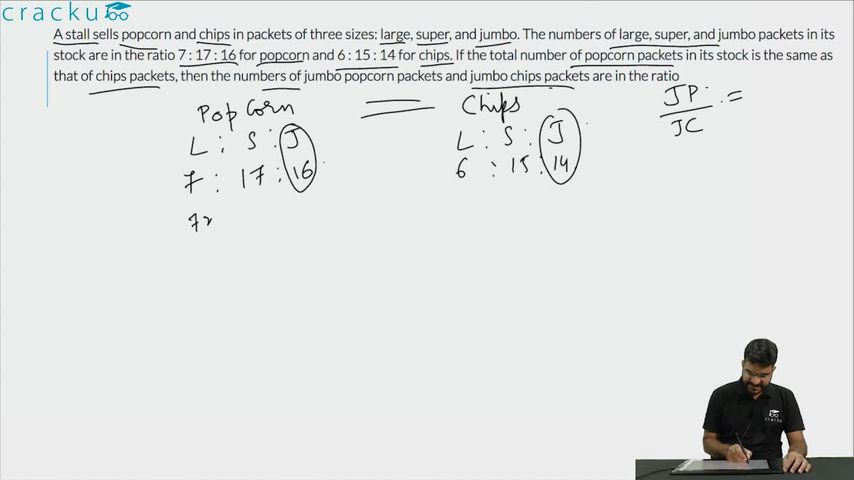Question 76

# A stall sells popcorn and chips in packets of three sizes: large, super, and jumbo. The numbers of large, super, and jumbo packets in its stock are in the ratio 7 : 17 : 16 for popcorn and 6 : 15 : 14 for chips. If the total number of popcorn packets in its stock is the same as that of chips packets, then the numbers of jumbo popcorn packets and jumbo chips packets are in the ratio

Solution

The ratio of L, S, J for popcorn = 7 : 17 : 16

Let them be 7$$x$$, 17$$x$$ and 16$$x$$

The ratio of L, S, J for chips = 6 : 15 : 14

Let them 6$$y$$, 15$$y$$ and 14$$y$$

Given, 40$$x$$ = 35$$y$$, $$x = \frac{7y}{8}$$

Jumbo popcor = 16$$x$$ = 16 * $$\frac{7y}{8}$$= 14$$y$$

Hence, the ratio of jumbo popcorn and jumbo chips = 1 : 1

### View Video Solution